Courses

# Previous Year Questions - Coordinate Geometry Class 10 Notes | EduRev

## Class 10 : Previous Year Questions - Coordinate Geometry Class 10 Notes | EduRev

The document Previous Year Questions - Coordinate Geometry Class 10 Notes | EduRev is a part of the Class 10 Course Mathematics (Maths) Class 10.
All you need of Class 10 at this link: Class 10

Short Answer Type Questions

Q.1. Find the coordinates of a point A, where AB is diameter of a circle whose centre is (2, -3) and B is the point (1, 4).    [Delhi 2019]
Ans
. AB is diameter of the circle.
Let C be centre of circle, coordinates of C are (2, -3).
So, C is mid-point of AB (diameter).
Let coordinates of A are (x, y).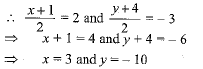∴ the coordinates of A are (3, - 10)

Q.2. Write the coordinates of a point P on x-axis which is equidistant from the points A (- 2, 0) and B(6, 0). [CBSE, Allahabad 2019]
Ans. Let coordinates of the P are (x, 0)
ATQ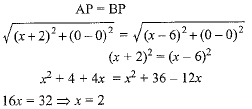∴ Coordinates of the point P are (2, 0).

Q.3. Find the coordinates of a point A, where AB is a diameter of the circle with centre (-2,2) and B is the point with coordinates (3, 4).     [CBSE 2019 (30/1/2)]
Ans. Let co-ordinate of point A be (x, y)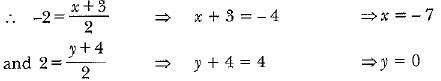∴ Co-ordinate of A are (-7,0)

Q.4. The mid point of the line segment joining A (2a, 4) and B (-2, 36) is (1, 2a + 1). Find the values of a and b.     [CBSE 2019 (30/5/11)]
Ans.
As (1, 2a + 1) is the mid point of AB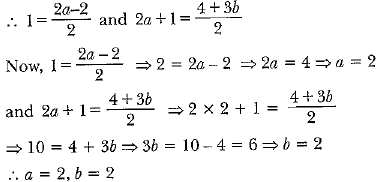Q.5. Find the distance of a point P(x, y) from the origin. [CBSE 2018]
Ans. Let the coordinates of the origin be O(0, 0)
By using distance formula,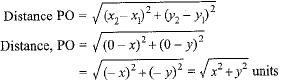Q.6. Find the linear relation between x and y such that P(x, y) is equidistant from the points A (1, 4) and B (-1, 2).    [CBSE 2018 (C)]
Ans.  P(x, y) is equidistant from the points A (1, 4) and B (- 1, 2)
PA = PB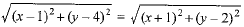Squaring both sides, we get
(x - 1)2 + (y - 4)2 = (x + 1)2 + (y - 2)2
x2 - 2x + 1 + y2 - 8y + 16 = x2 + 2x + 1 + y2 - 4y + 4
-2x + 17 - 8y = 2x - 4y + 5
- 4x - 4y = -12
x + y = 3

Q.7. A(5, 1); B(1, 5) and C(-3, - 1) are the vertices of ΔABC. Find the length of median AD.    [CBSE 2018 (C)]
Ans. AD is median of ΔABC
∴ D is mid-point of BC
Coordinates of D are: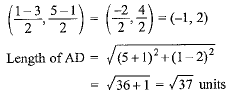Q.8. Find the ratio in which P(4, m) divides the line segment joining the points A(2, 3) and B(6, - 3). Hence find m.    [CBSE 2018]
Ans.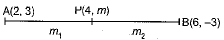Let, p(4, m) divides A(2, 3) and B(6, - 3) in the ratio m1 : m2,
[By using section formula,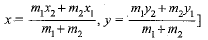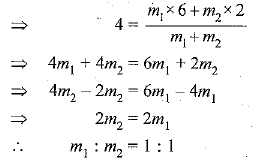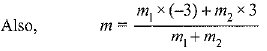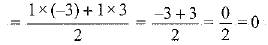∴ m = 0

Q.9. If A (-2 , 1), B(a, 0), C(4, b) and D (1, 2) are the vertices of a parallelogram ABCD, find the values of a and b. Hence find the lengths of its sides.    [CBSE 2018]
Ans.  We know, the diagonals of a parallelogram bisect each other at a point. Let they bisect at O, then by using mid-point formula for diagonal AC,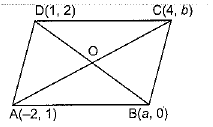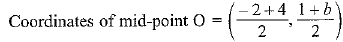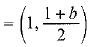Also,for diagonal BD,
Coordinates of mid-point O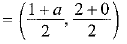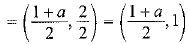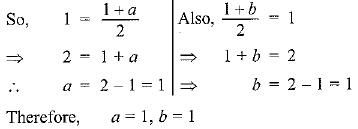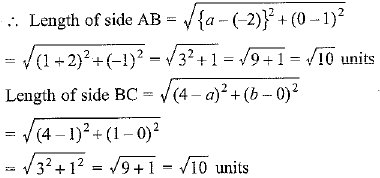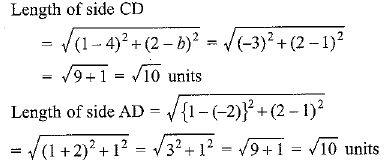Q.10. If coordinates of two adjacent vertices of a parallelogram are (3,2), (1,0) and diagonals bisect each other at (2, -5), find coordinates of the other two vertices. [CBSE 2018 (C)]
Ans. Let ABCD be a parallelogram, diagonals AC and BD intersects at O.
Let A(3, 2), B (1, 0) and O (2, - 5) are coordinates.
Let coordinates of C are (a, b), and coordinates of D are (x, y). As diagonals of parallelogram bisect each other at O. So, O is mid point of AC and BD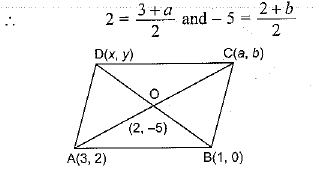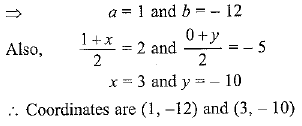Q.11. If A (-5, 7), B (-4, - 5), C (-1, - 6) and D(4, 5) are the vertices of a quadrilateral, find the area of the quadrilateral ABCD.    [CBSE 2018]
Ans.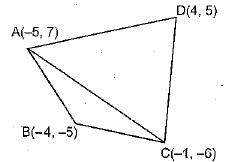Join AC.AC divides quadrilateral ABCD into ΔABC and ΔACD.
By using formula,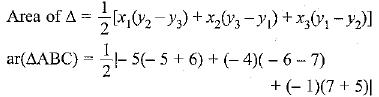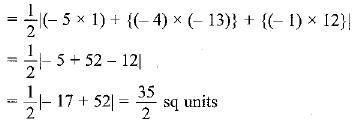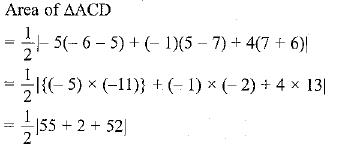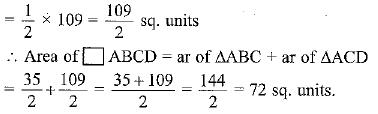Q.12. If the area of triangle with vertices (x, 3), (4, 4) and (3, 5) is 4 square units, find x.    [CBSE 2018 (C)]
Ans. If (x1, y1) (x2, y2)(x3, y3) be the vertices of the triangle then area of triangle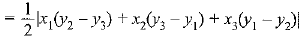If coordinates are (x, 3), (4, 4) and (3, 5), then area of triangle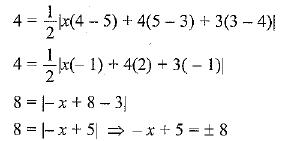- x + 5 = 8 or - x + 5 = - 8
- x = 3 or - x = - 13
x = - 3 or x = 13

Q.13. Show that ΔABC, where A(-2, 0), B(2, 0), C(0, 2) and ΔPQR where P(-4, 0), Q(4, 0), R(0, 4) are similar triangles,     [CBSE Delhi 2017]
OR
Show that ΔABC with vertices A(-2, 0), B(0, 2) and C(2, 0) is similar to ΔDEF with vertices D (-4, 0), F(4, 0) and E(0, 4).     [NCERT Exemplar, CBSE (F) 2017]
Ans.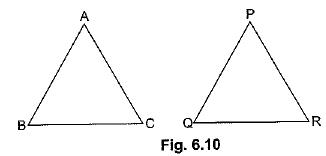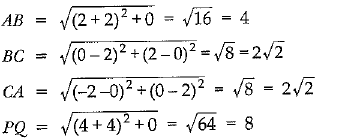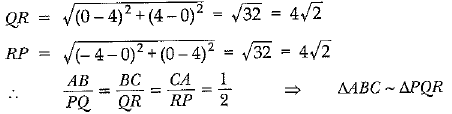Q.14. If the distance between the points (4, k) and (1,0) is 5, then what can be the possible values of k ?     [Delhi 2017]
Ans.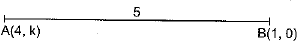Using distance formula,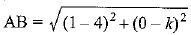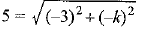⇒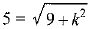On squaring, we get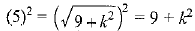⇒ 25 - 9 = k2  ⇒ k2 = 16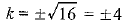Q.15. If the point (x, y) is equidistant from the points (a + b, b - a) and (a - b, a + b), prove that bx = ay.    [AI 2017 (C)]
Ans. Consider that point P(x, y) is equidistant from A (a + b, b - a) and B (a - b, a + b)
∴ PA = PB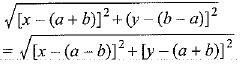Squaring both sides, we get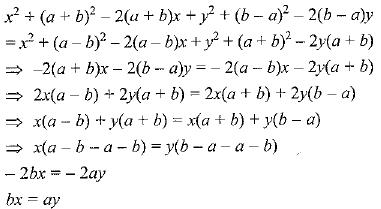Q.16. Find the area of the triangle formed by joining the mid points of the sides of the triangle whose vertices are (0, -1), (2,1) and (0, 3). [AI 2017(C)]
Ans. Let D, E and F are mid-points of sides BC, CA and AB respectively of ΔABC.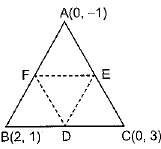Area of ΔABC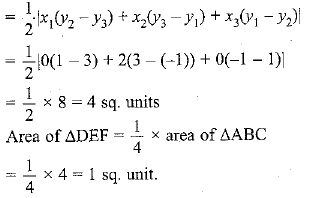Q.17. If two adjacent vertices of a parallelogram are (3, 2) and (- 1, 0) and the diagonals intersect at (2, - 5), then find the coordinates of the other two vertices.     [CBSE (F) 2017]
Ans. Let other two coordinates are (x, y) and (x y').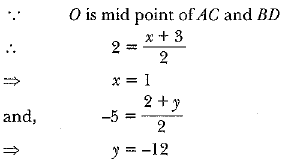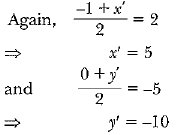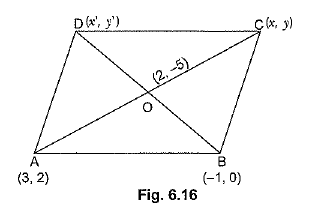Hence, co-ordinates are (1, -12) and (5, -10).

Q.18. Find the coordinates of a point on the x-axis which is equidistant from the points A(2, -5) and B(-2, 9).    [CBSE Delhi 2017 (C)]
Ans.
Let P(x, 0) be any point on x-axis.
Now, P(x, 0) is equidistant from point A(2, -5) and B(-2, 9)
∴ AP = BP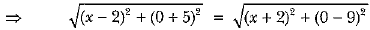Squaring both sides, we have
(x - 2)2 + 25 = (x + 2)2 + 81
⇒ x2 + 4 - 4x + 25 = x2 + 4 + 4x + 81 ⇒ - 8x = 56
∴ x = 56/-8 = -7
∴ The point on the x-axis equidistant from given point is (- 7, 0).

Q.19.  Find the values of ft if the points A (k + 1, 2k), B(3k, 2k + 3) and C(5k - 1, 5k) are collinear.    [NCERT Exemplar, CBSE (AI) 2017]
Ans. ∵ Points A(k + 1, 2k), B (3k, 2k + 3) and C (5k - 1, 5k) are collinear.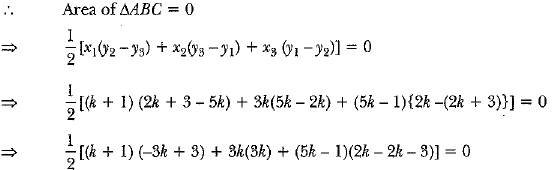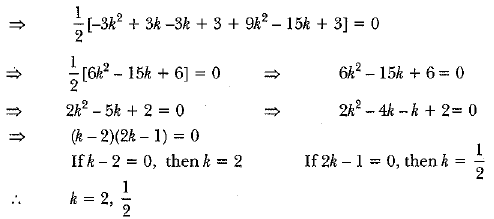Q.20. In the given figure, ΔABC is an equilateral triangle o f side 3 units. Find the coordinates of the other two vertices.    [CBSE (F) 2017]
Ans.  Since AB = BC = AC = 3 units
∴ Co-ordinates of B are (5, 0)
Let co-ordinates of C be (x.y)
AC2 = BC2    [∵ΔABC is an equilateral triangle]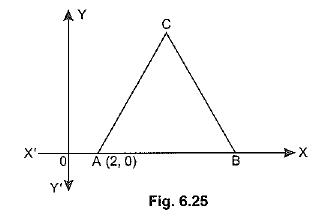Using distance formula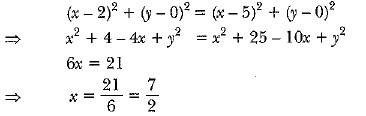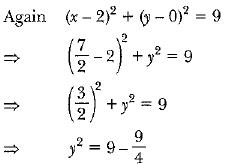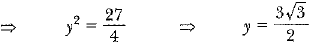(+ ve sign to be taken)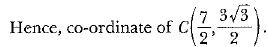Q.21. The area of a triangle is 5 sq units. Two of its vertices are (2, 1) and (3, -2). If the third vertex is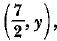find the value of y,      [CBSE Delhi 2017]
Ans.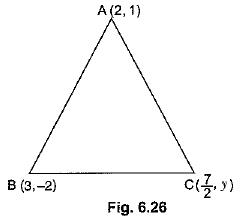Given: ar(ΔABC) = 5 sq. units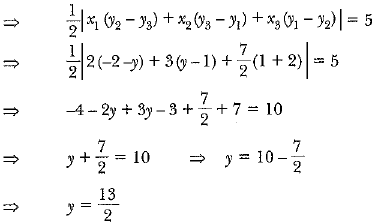Q.22. If a ≠ b 0, prove that the points (a, a2) (b, b2), (0, 0) will not be collinear.     [CBSE Delhi 2017]
Ans. ∵ We know that three points are collinear if area of triangle = 0.
∴ Area of triangle with vertices (a, a2), (b, b2) and (0, 0)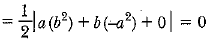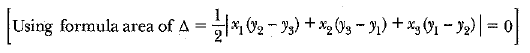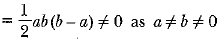∵ Area of A ≠ 0
∴  Given points are not collinear.

Q.23. The coordinates o f the points A , B and C are (6 , 3) , (-3 ,5) and (4, -2) respectively. P(x, y) is any point in the plane.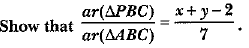[CBSE (F) 2016]
Ans.  Given points are P(x,y), B(-3, 5), C(4, -2), A(6, 3)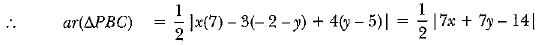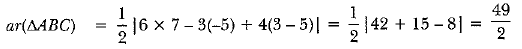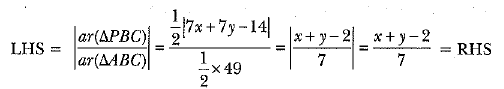Q.24. If the point P(x, y) is equidistant from the points A (a + b, b - a) and B(a - b, a + b). Prove that bx = ay.     [CBSE(AI) 2016]
Ans. Given, PA = PB or (PA)2 = (PB)2
(a + b - x)2 + (b - a - y)2 = (a - b - x)2  + (a + b - y)2
⇒ (a + b)2 + x2 - 2ax - 2bx + (b - a)2 + y2 - 2by + 2ay
= (a - b)2 + x2 - 2ax + 2bx + (a + b)2 + y2 - 2ay - 2by
⇒ 4ay = 4bx  or  bx = ay
Hence proved.

Q.25.  If the point C (-1, 2) divides internally the line segment joining the points A (2, 5) and B(x, y) in the ratio of 3 : 4, find the value of x2 + y2.    [CBSE (F) 2016]
Ans.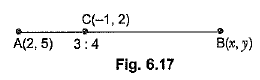∵ C (-1, 2) divides AB in the ratio 3 : 4.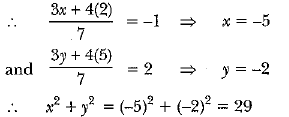Q.26. Prove that the area of a triangle with vertices (t, t - 2) , (t + 2, t + 2) and (t + 3, t) is independent of t.     [CBSE Delhi 2016]
Ans.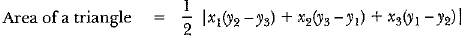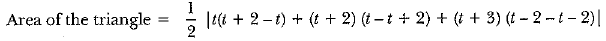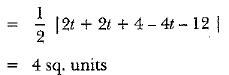which is independent of t.
Hence proved.

Q.27. Find the ratio in which 3-axis divides the line segment joining the points A(5, - 6) and 5 (-1, -4). Also find the coordinates of the point of division.     [CBSE Delhi 2016]
Ans.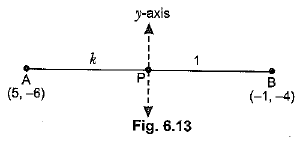Let the point on y-axis be p (0, y) and AP : PB = k : 1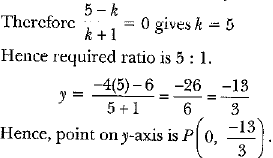Q.28.  Let P and Q be the points of trisection of the line segment joining the points A(2, - 2) and 5(-7, 4) such that P is nearer to A. Find the coordinates of P and Q.     [CBSE (AI) 2016]
Ans.  ∵ P divides AB in the ratio 1 : 2.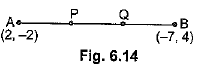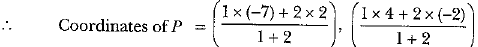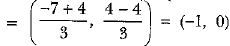∵ Q is the mid-point of PB.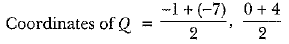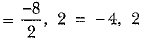Q.29. Find the ratio in which the point (-3, k) divides the line-segment joining the points (-5, -4) and (-2, 3). Also find the value of k.     [CBSE (F) 2016]
Ans. Let Q, divide AB in the ratio of p : 1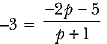⇒  -3p - 3 = -2p - 5 ⇒ p = 2
∴ Ratio is 2:1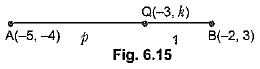Q.30. Show that the points A (a, a), B (- a, - a) and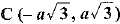form an equilateral triangle.    [Foreign 2015]
Ans.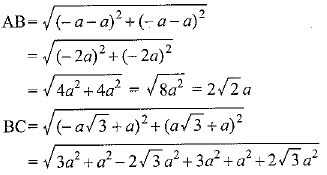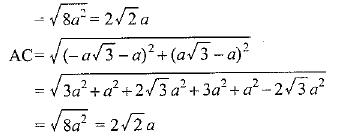Since AB = BC = AC.    ∴ ΔABC is equilateral.

Long Answer Type Questions

Q.1.  Find the area of a quadrilateral ABCD whose vertices are A(1, 1), B(7, -3), C(7, 21) and D(7, 21)     [AI 2017(C)]
Ans. ar(quad. ABCD) = ar(ΔABC) + ar(ΔADC)    ...(i)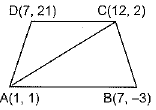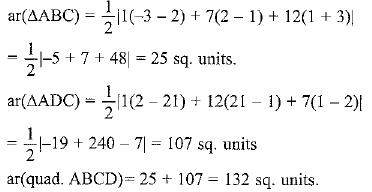Q.2. In Fig. 6.32, the vertices of ΔABC are A(4, 6), B (1, 5) and C(7, 2). A line-segment DE is drawn to intersect the sides AB and AC at D and E respectively such that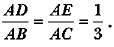Calculate the area of ΔADE and compare it with area of ΔABC.    [CBSE (AI) 2016]
Ans.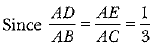⇒ D and E divide AB and AC respectively in the ratio 1 : 2.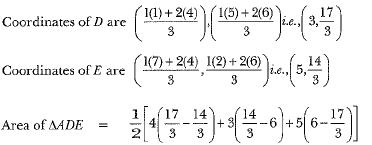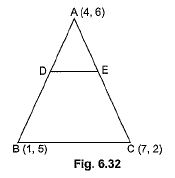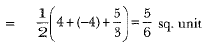Area of ΔABC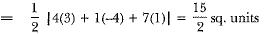Area of ΔADE : area oF ΔABC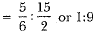Q.3. The base BC of an equilateral triangle ABC lies on 3-axis. The coordinates of point C are (0, -3). The origin is the mid-point of the base. Find the coordinates of the points A and B. Also find the coordinates of another point D such that BACD is a rhombus.     [CBSE (F) 2015]
Ans. ∵ O is the mid-point of the base BC.
∴ Coordinates of point B are (0, 3).
So, BC = 6 units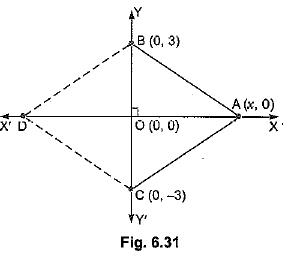Let the coordinates of point A be (x, 0).
Using distance formula,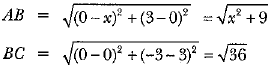Also,   AB = BC (∵ ΔABC is an equilateral triangle)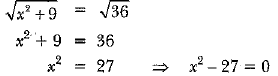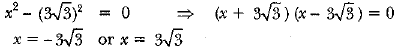⇒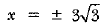∴ Coordinates of points = (x, 0) =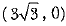Since BACD is a rhom bus.
∴ AB = AC = CD = DB
∴ Coordinates of point D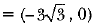Offer running on EduRev: Apply code STAYHOME200 to get INR 200 off on our premium plan EduRev Infinity!

## Mathematics (Maths) Class 10

54 videos|346 docs|103 tests

,

,

,

,

,

,

,

,

,

,

,

,

,

,

,

,

,

,

,

,

,

;